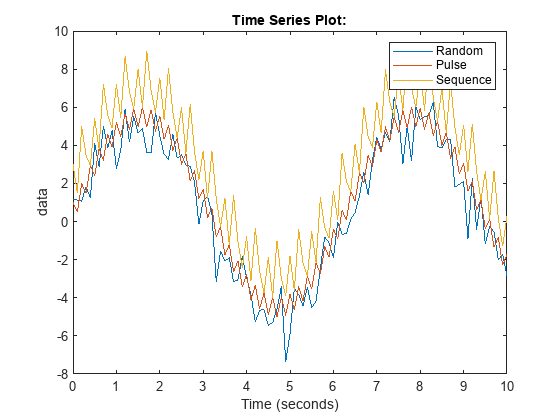# How to Create a Mask Popup Parameter that can be tuned in Rapid Accelerator

A few weeks ago I published this blog post: Getting the most out of Rapid Accelerator mode – Version R2023b, where I described how to simulate a model multiple times in Rapid Accelerator mode for maximum performance using the parameter RapidAcceleratorUpToDateCheck='off'.
In this previous post, I was tuning the value of a Gain block. This week I received the question: Can I do the same for a parameter of type popup?

## The Problem

I have this model with a masked subsystem that has a popup parameter. Under the mask, the variable associated with the popup, choice, is used to control what type of noise is added to the signal: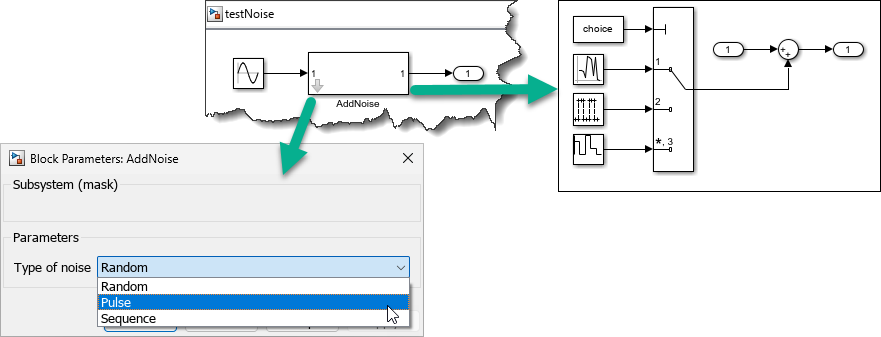If I try to simulate the model as described in the previous blog post, I get an error saying that it's not possible to use setBlockParameters and RapidAcceleratorUpToDateCheck='off' at the same time:
mdl = 'testNoise';
for i = 1:3
in(i) = in(i).setModelParameter(SimulationMode='Rapid');
in(i) = in(i).setModelParameter(RapidAcceleratorUpToDateCheck='off');
end
try
in(1) = in(1).setBlockParameter(blk,'choice','Random');
catch ME
ME.message
end
ans = 'Cannot set 'BlockParameters' on SimulationInput object when 'RapidAcceleratorUpToDateCheck' is set to 'off'.'

## The Solution

The solution to this is described in the documentation page Tune Mask Enumeration Parameters - Popup and Radio Button. I find this page is a bit long and complex, so I will try to summarize the process here.
The first thing you need to do is associate an enumeration with the popup parameter. For this example, the enumeration is:
enumeration
Random (1,'Random')
Pulse (2,'Pulse')
Sequence (3,'Sequence')
end
end
I then associate it with the parameter in the Mask Editor by double-clicking on the Type options field: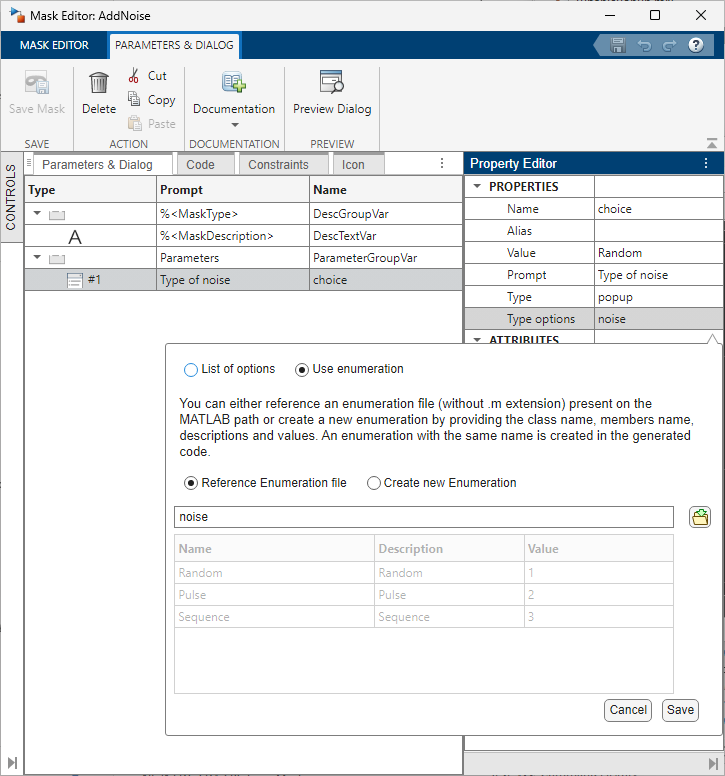In the Subsystem under the mask, I need to modify how the variable is specified in the block dialog. For this example, instead of using the variable choice directly, I need to specify noiseValues(choice). The documentation explains the syntax, but in short you begin with the enumeration name (noise in this example) and you append "Values" to it. Then you pass to it, between parenthesis, the popup variable (choice in this example):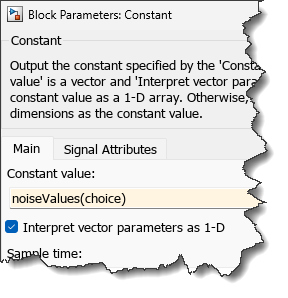If you create the masked Subsystem that way, you will notice that the dialog has a new "Associate Variable" option: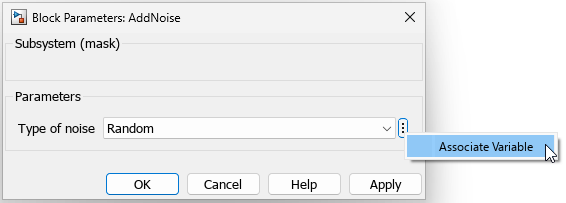This will open a dialog to specify the variable and optionally create it if it does not exist. For this example, I will create it myself:
noiseType = noise.Random;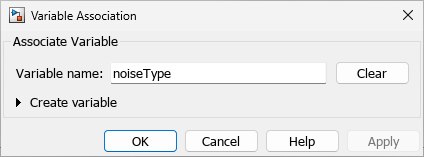Once you click ok, in the block dialog, you will see the variable name and the enumeration type next to it: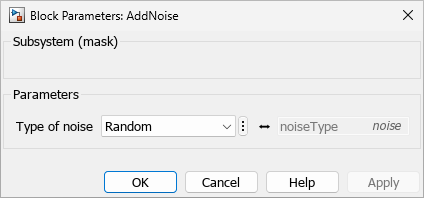And that's it, now it's time to simulate the model:
mdl = 'testNoiseTunable';
for i = 1:3
in(i) = in(i).setModelParameter(SimulationMode='Rapid');
in(i) = in(i).setModelParameter(RapidAcceleratorUpToDateCheck='off');
end
in(1) = in(1).setVariable('noiseType',noise.Random);
in(2) = in(2).setVariable('noiseType',noise.Pulse);
in(3) = in(3).setVariable('noiseType',noise.Sequence);
out = sim(in,'ShowProgress','off');
Once the simulation completes, we can plot the results and confirm that the parameter has been tuned:
plot(out(1).yout{1}.Values);
hold on
plot(out(2).yout{1}.Values);
plot(out(3).yout{1}.Values);
hold off
legend({'Random','Pulse','Sequence'});